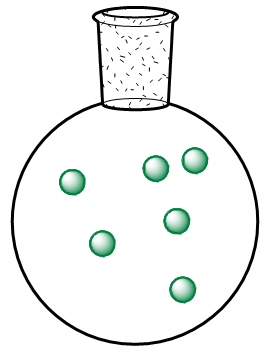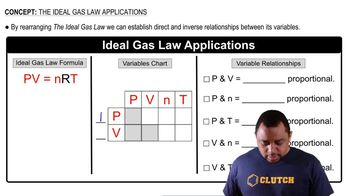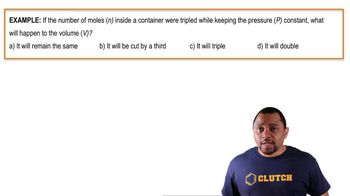Start typing, then use the up and down arrows to select an option from the list.
1:47 minutes
Problem 3
Textbook Question

# Consider the sample of gas depicted here. What would the drawing look like if the volume and temperature remained constant while you removed enough of the gas to decrease the pressure by a factor of 2? (a) It would contain the same number of molecules. (b) It would contain half as many molecules. (c) It would contain twice as many molecules. (d) There is insufficient data to say.Verified Solution
This video solution was recommended by our tutors as helpful for the problem above.
138views